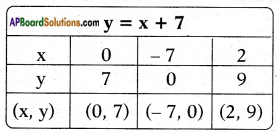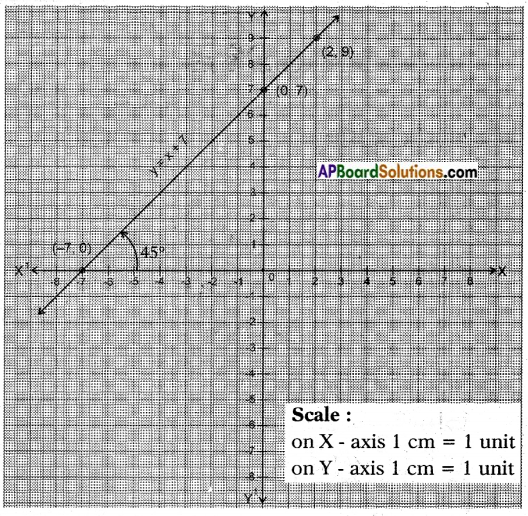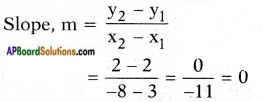## AP State Syllabus SSC 10th Class Maths Solutions 7th Lesson Coordinate Geometry InText Questions

AP State Board Syllabus AP SSC 10th Class Maths Textbook Solutions Chapter 7 Coordinate Geometry InText Questions and Answers.

### 10th Class Maths 7th Lesson Coordinate Geometry InText Questions and Answers

Do these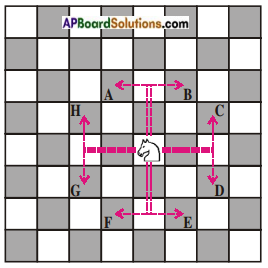Question 1.
From the figure write coordinates of the points A, B, C, D, E, F, G, H.          (Page No. 159)
Given: Knight is at the origin (0, 0).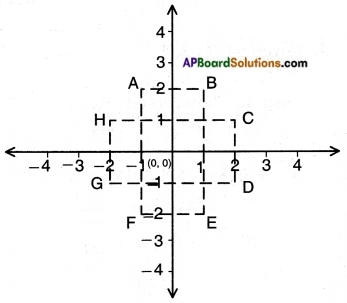Therefore, A (- 1, 2), B (1, 2), C (2, 1), D (2, – 1), E (1, – 2), F (-1, -2), G (-2, -1) and H (-2, 1).Question 2.
Find the distance covered by the Knight in each of its 8 moves i.e., find the distance of A, B, C, D, E, F, G and H from the origin.    (Page No. 159)
Origin (0, 0).
Points A, B, C, D, E, F, G and H.
Distance of any point P(x, y) from the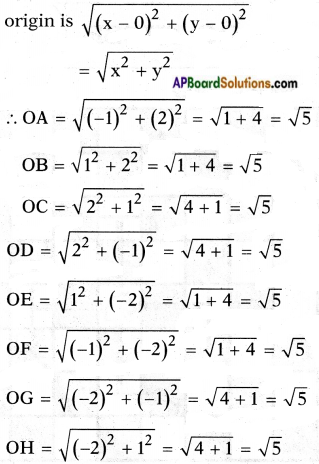Question 3.
What is the distance between two points H and C? And also find the distance between two points A and B.        (Page No. 159)
Given: H (- 2, 1), C (2, 1), A (- 1, 2), B (1, 2).
Distance between any two points P(x1, y1) and Q(x2, y2) is
$$\overline{\mathrm{PQ}}$$ = $$\sqrt{\left(x_{2}-x_{1}\right)^{2}+\left(y_{2}-y_{1}\right)^{2}}$$
∴ Distance between H and C is HC
= $$\sqrt{[2-(-2)]^{2}+(1-1)^{2}}$$
= $$\sqrt{4^{2}+(0)^{2}}$$
= $$\sqrt{16}$$
= 4 units
Distance between A and B is
AB = = $$\sqrt{[1-(-1)]^{2}+(2-2)^{2}}$$
= $$\sqrt{2^{2}+0^{2}}$$
= $$\sqrt{4+0}$$
= 2 units
[!! H, C are points on a line parallel to X – axis.
∴ HC = |x2 – x1| = |2 – (- 2)| = 4 Similarly,
AB = |x2 – x1| = |-1-1| = 2 ]Question 4.
Where do these following points lie (-4, 0), (2, 0), (6, 0), (-8, 0) on coordinate plane?    (Page No. 160)
Given points are (- 4, 0), (2, 0), (6, 0), (- 8, 0).
All these points have their y-coordinates = 0
∴ These points lie on X-axis.

Question 5.
What is the distance between points (- 4,0) and (6, 0) on coordinate plane?    (Page No. 160)
Given points = (- 4, 0) and (6, 0).
These two points lie on the X – axis.
∴ Distance between them = |x2 – x1| = |16 – (-4)| = 16 + 41 = 10 units.

Question 6.
Find the distance between the following pairs of points:    (Page No. 162)
i) (3, 8), (6, 8).
Given points = A (3, 8) and B (6, 8)
These two points lie on the line parallel to X – axis.
Distance between A (3, 8) and B (6, 8) = |x2 – x1|
= |6 – 3| = 3 units.

ii) (-4, -3), (-8, -3).
Given points = A (- 4, – 3) and B (- 8, – 3)
These two points lie on the line parallel to X – axis.
∴ Distance between A (- 4, – 3) and B (- 8, – 3) = |x2 – x1|
= |-8 – (-4)|
= |-8 + 4|
= |-4| = 4 units.

iii) (3, 4), (3, 8).
Given points = A (3, 4) and B (3, 8) These two points lie on the line parallel to Y – axis.
∴ Distance between A (3, 4) and B (3, 8)= |y2 – y1|
= |8 – 4| = 4 units.

iv) (-5, -8), (-5, -12).
Given points = A (-5, -8) and B (-5, -12)
These two points lie on the line parallel to Y – axis.
∴ Distance between A (-5, -8) and B (-5, -12) = |y2 – y1|
= |-12 – (-8)|
= |-12 + 8|
= |-4| = 4 units.Question 7.
Find the distance between the following points.    (Page No. 162)
i) A = (2, 0) and B(0, 4)
Given points = A (2, 0) and B (0, 4)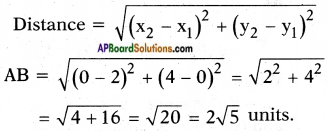ii) P(0, 5) and Q(12, 0).
Given points = P (0, 5) and Q (12, 0)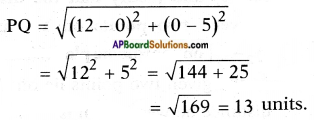Question 8.
Find the distance between the following pair of points.    (Page No. 164)
i) (7, 8) and (- 2, 3)
Given points = (7, 8) and (- 2, 3)
Distance formula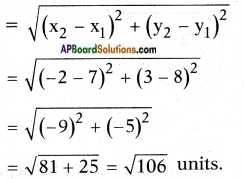ii) (- 8, 6) and (2, 0)
Given points = (- 8, 6) and (2, 0).
Distance formula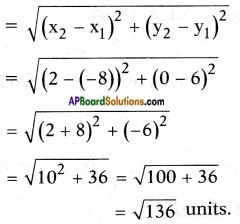Try these

Question 1.
Where do these following points lie – (0, -3), (0, -8), (0, 6), (0, 4) on coordinate plane?    (Page No. 161)
As the x – coordinate of all these points is zero, all points lie on Y – axis.

Question 2.
What is the distance between (0, -3), (0, -8) and justify that the distance between two points on Y – axis is |y2 – y1| on coordinate plane?    (Page No. 161)
As the given two points lie on Y-axis, distance between them is
|y2 – y1| = |-3 + 8| = |5| = 5 units.
Let (0, y1) and (0, y2) be any two points on Y-axis, then distance between them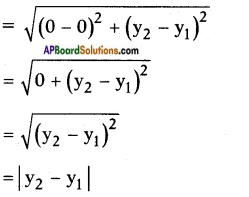[∵ distance can’t be negative]

Question 3.
Find the distance between points ‘O’(origin) and ‘A’ (7, 4).    (Page No. 162)
Given: Origin and a point (7, 4).
Distance of a point (x, y) from the origin is $$\sqrt{x^{2}+y^{2}}$$
= $$\sqrt{7^{2}+4^{2}}$$ = $$\sqrt{49+16}$$ = √65 units.

Question 4.
Find the distance between A(1, -3) and B(-4, 4) and rounded to two decimals.      (Page No. 164)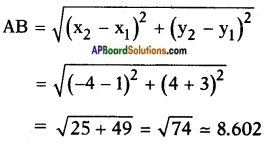Think & DiscussQuestion 1.
How will you find the distance between two points in which x or y coordinates are same but not zero?  (Page No. 161)
Let the points be A(2, 3), B(2, 5).
Here the x-coordinates are same, then the distance between the points A and B is |y2 – y1| = |5 – 3| = 2 units.
If the points are P (4, 3), Q (- 8, 3),
here the y-coordinates are same. In such a case, the distance is given by |x2 – x1| = |-8-4| = |-12|
= 12 units.
i.e.,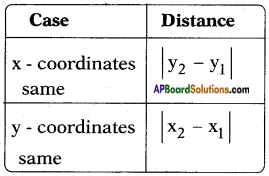Question 2.
Ramu says the distance of a point P(x, y) from the origin O(0, 0) is $$\sqrt{x^{2}+y^{2}}$$. Do you agree with Ramu or not? Why?    (Page No. 163)
Yes. The distance between O(0, 0) and
P(x, y) is $$\sqrt{(x-0)^{2}+(y-0)^{2}}$$
= $$\sqrt{x^{2}+y^{2}}$$

Question 3.
Ramu also writes the distance formula as AB = $$\sqrt{\left(x_{2}-x_{1}\right)^{2}+\left(y_{2}-y_{1}\right)^{2}}$$. Why?    (Page No. 163)
(x1 – x2)2 is same as (x2 – x1)2
and (y1 – y2)2 is same as (y2 – y1)2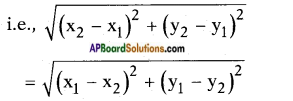Question 4.
Sridhar calculated the distance between T(5, 2) and R(-4, -1) to the nearest tenth is 9.5 units. Now you find the distance between P (4, 1) and Q (-5, -2). Do you get the same answer that Sridhar got? Why?    (Page No. 164)
Given points (4, 1), (-5, -2)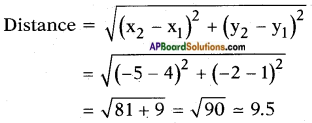∵ (x)2 = (-x)2
(x1 – x2)2 = (x2 – x1)2 or
The given points (4, 1) and (-4, -1) are images to each other and (5, 2), (-5, -2) are also images to each other.Do these

Question 1.
Find the point which divides the line segment joining the points (3, 5) and (8, 10) internally in the ratio 2 : 3.  (Page No. 171)
Let P (x, y) be the required point then, P (x, y) =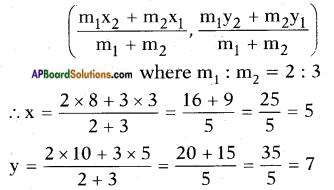∴ P (x, y) = (5, 7)

Question 2.
Find the midpoint of the line segment joining the points (2, 7) and (12, -7).  (Page No. 171)
Midpoint of the line joining the points (x1, y1) and (x2, y2) is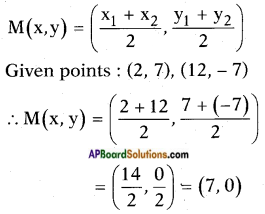Question 3.
Find the centroid of the triangle whose vertices are (-4, 6), (2, -2) and (2, 5) respectively.    (Page No. 173)
Given points: (- 4, 6), (2, – 2) and (2,-5).
The coordinates of the centroid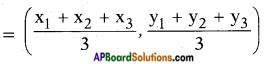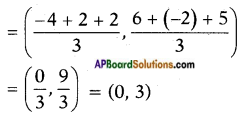∴ the centroid is (0, 3)Question 4.
Find the trisectional points of line joining (2, 6) and (-4, 8).  (Page No. 175)
A (2, 6) and B (- 4, 8) be the given points.
Let P, Q divide the line joining of $$\overline{\mathrm{AB}}$$ in the ratio 1 : 2 and 2 : 1.
Section formula (x, y)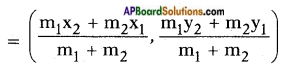For P(x, y) the ratio is 1 : 2.
P(x,y).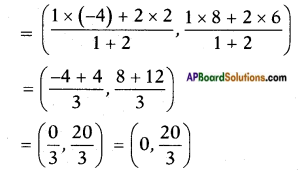For Q (x, y) the ratio is 2 : 1.
Q (x, y)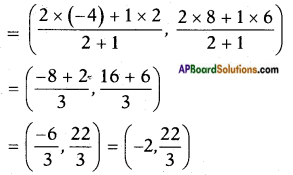∴ The points of trisection are $$\left(0, \frac{20}{3}\right)$$ and $$\left(-2, \frac{22}{3}\right)$$.

Question 5.
Find the trisectional points of line joining (-3, -5) and (-6, -8).  (Page No. 175)
Given: A (- 3, – 5) and B (- 6, 8).
Let P and Q be the points of trisection of $$\overline{\mathrm{AB}}$$, then P divides $$\overline{\mathrm{AB}}$$ in the ratio 1 : 2.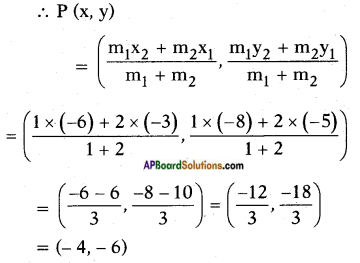Q (x, y) divides $$\overline{\mathrm{AB}}$$ in the ratio 2 : 1
Q (x, y)
∴ The points of trisection are P (-4, -6) and Q (-5, -7).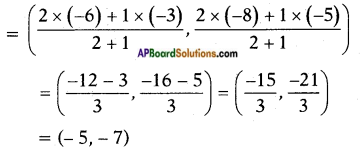Try these(Page No. 172)

Let A(4,2), B(6, 5) and C(l, 4) be the vertices of △ABC.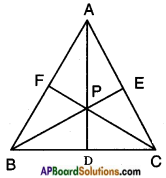Question 1.
The median from A meets BC at D. Find the coordinates of the point D.
D is the midpoint of BC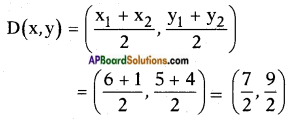Question 2.
Find the coordinates of the point P on AD such that AP : PD = 2 : 1.      (Page No. 172)
P is a point on AD which divides AD in the ratio 2 : 1
∴ P(x,y)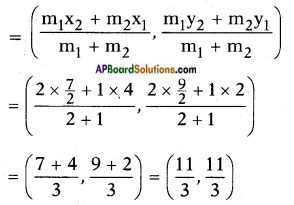Midpoint of the line joining the points (x1, y1) and (x2, y2) is

Question 3.
Find the points which divide the line segment BE in the ratio 2 : 1 and also that divide the line segment CF in the ratio 2 : 1.    (Page No. 172)
Given: B (6, 5), E$$\left(\frac{5}{2}, 3\right)$$
Let it be P(x, y) =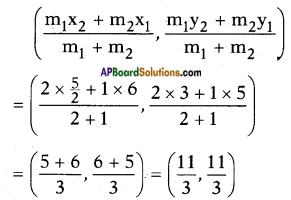Similarly, let P divide C(1, 4) and F$$\left(5, \frac{7}{2}\right)$$ in the ratio 2:1.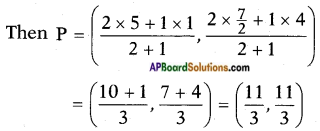Question 4.
What do you observe? Justify the point that divides each median in the ratio 2 : 1 is the centriod of a triangle.    (Page No. 172)
From the above problems, we conclude that the point ‘P’ divides each median in the ratio 2:1.
i.e., the three medians are concurrent at P, which is called the centroid.
A centriod divides each median in the ratio 2 : 1.Question 5.
The points (2, 3) (x, y) (3, -2) are the vertices of a triangle. If the centroid of this triangle is origin then find (x, y).   (Page No. 173)
Given vertices of triangle are (2, 3) (x, y) (3, -2)
now formula for centroid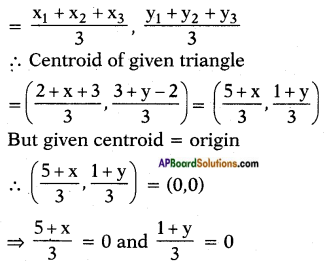∴ 5 + x = 0 and 1 + y = 0
⇒ x = -5 and y = -1
∴ (x, y) = (-5, -1)

Think & Discuss

(Page No. 174)

Question 1.
The line joining points A(6, 9) and B(-6, -9) are given.
a. In which ratio does origin divide $$\overline{\mathrm{AB}}$$? And what it is called for $$\overline{\mathrm{AB}}$$?
Given : A (6, 9), B (-6, -9)
Let origin O(0, 0) divides $$\overline{\mathrm{AB}}$$ in the ratio k : 1 internally.
[By section formula]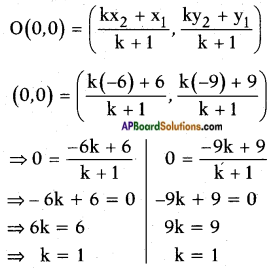The ratio is 1 : 1.
Here the origin bisects $$\overline{\mathrm{AB}}$$.
∴ Origin is called the midpoint of $$\overline{\mathrm{AB}}$$.

b. In which ratio does the point P(2, 3) divide $$\overline{\mathrm{AB}}$$?
Given: A (6, 9), B (-6, -9) and P (2, 3) divide $$\overline{\mathrm{AB}}$$ internally in the ratio say k : 1. [By section formula]
Then P (2, 3) =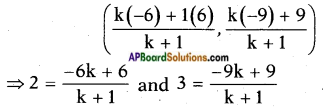⇒ 2k + 2 = -6k + 6 and 3k + 3 = -9k + 9
⇒ 8k = 6 – 2 and 3k + 9k = 9 – 3
⇒ k = $$\frac{4}{8}$$ and 12k = 6
⇒ k = $$\frac{1}{2}$$ and k = $$\frac{6}{12}$$
⇒ k = $$\frac{1}{2}$$
∴ The ratio (k : 1) = $$\left(\frac{1}{2}: 1\right)$$ = 2 : 1

c. In which ratio does the point Q(-2, -3) divide $$\overline{\mathrm{AB}}$$?
Let Q divide $$\overline{\mathrm{AB}}$$ in the ratio say k : 1 internally, then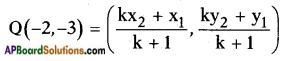[By section formula]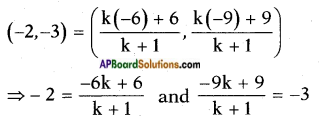⇒ 6k + 6 = -2k-2 and -9k + 9 = -3k-3
⇒ -6k + 2k = -2-6 and -9k + 3k = -3-9
⇒ -4k = -8 and -6k = -12
⇒ k = $$\frac{-8}{-4}$$ = 2 and k = $$\frac{-12}{-6}$$ = 2
∴ The ratio is k : 1 = 2 : 1

d. Into how many equal parts is $$\overline{\mathrm{AB}}$$ divided by P and Q?
Since P, Q divide $$\overline{\mathrm{AB}}$$ in the ratio 1 : 2 and 2 : 1, $$\overline{\mathrm{AB}}$$ is divided into three equal parts by P and Q.

e. What do we call P and Q for $$\overline{\mathrm{AB}}$$?
P and Q are the points of trisection of $$\overline{\mathrm{AB}}$$.Do these

Question 1.
Find the area of the triangle whose vertices are
(5, 2) (3,-5) and (-5,-1).  (Page No. 180)
Given: The vertices of the triangle are (5, 2), (3, -5) and (-5, -1).
Area of the triangle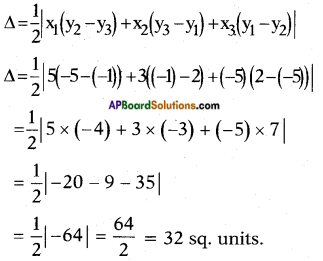Question 2.
(6, -6), (3, -7) and (3, 3).    (Page No. 180)
Given: The vertices of a*triangle are (6, -6), (3, -7) and (3, 3).
Area of a triangle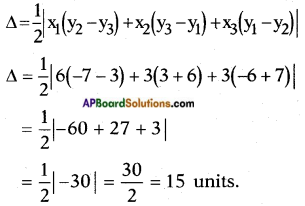Question 3.
Verify whether the following points are collinear or not.  (Page No. 182)
i) (1, -1), (4, 1), (-2, -3).
Given: Three points (1, -1), (4, 1), (-2, -3).
Area of the triangle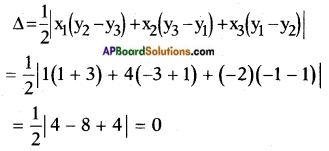As the area of the triangle is ‘O’, the three points are collinear.ii) (1, -1), (2, 3), (2, 0).
Given points are (1, -1), (2, 3), (2, 0).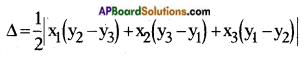Area of the triangle formed by the given three points is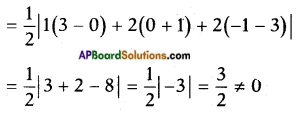△ ≠ 0.
Hence the points are not collinear.

iii) (1, -6), (3, -4), (4, -3).
The given points are (1, -6), (3, -4), (4, -3).
Area of a triangle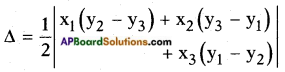Area of the triangle formed by the given three points is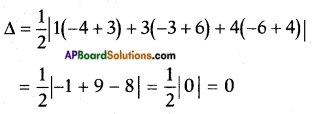As △ = 0, the points are collinear.

Question 4.
Find the area of the triangle whose lengths of sides are 15 m, 17 m, 21 m. (use Heron’s Formula)  (Page No. 183)
Given: The sides of a triangle
a = 15 m; b = 17 m and c = 21 m.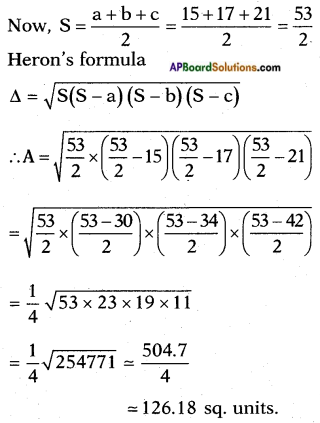Note: As the ‘height’ is not given we can’t verify the above answer by A = $$\frac{1}{2}$$bh

Question 5.
Find the area of the triangle formed by the points (0, 0), (4, 0), (4, 3) by using Heron’s formula.  (Page No. 183)
The given points are O (0, 0), A (4, 0) and B (4, 3).
Then the sides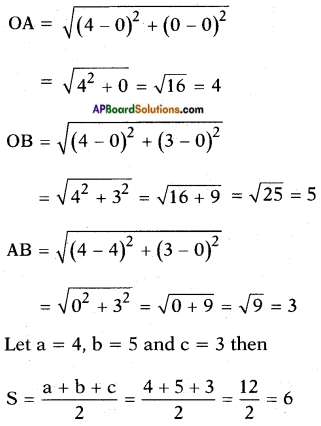Heron’s formula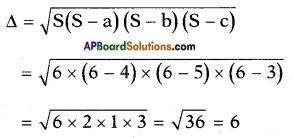Verification:
If the sides are 3, 4 and 5 units, clearly the triangle is a right triangle.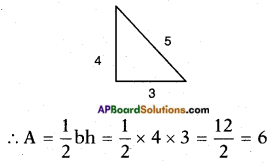Try these

Question 1.
Take a point A on X-axis and B on Y-axis and find area of the triangle AOB. Discuss with your friends what did they do.      (Page No. 178)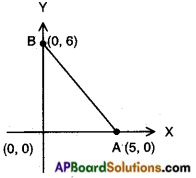[∴ Axes are perpendicular to each other]
Consider the points A(5, 0) and B(0, 6).
△AOB = $$\frac{1}{2}$$ × base × height
= $$\frac{1}{2}$$ × 6 × 5 = 15 sq. units.
Area of a triangle A (x, 0), O (0, 0) and
B (0, y) is $$\frac{1}{2}$$xy.

Question 2.
Find the area of the square formed by (0, -1), (2, 1), (0, 3) and (-2, 1) taken in order are as vertices.    (Page No. 178)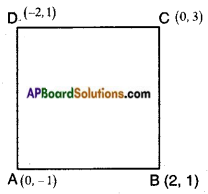Let A (0, -1), B (2, 1), C (0, 3) and D (- 2, 1) are the vertices of a square.
Area of the square ABCD = side2
= AB2
But, AB = $$\sqrt{\left(x_{2}-x_{1}\right)^{2}+\left(y_{2}-y_{1}\right)^{2}}$$
= $$\sqrt{(2-0)^{2}+(1+1)^{2}}$$
= $$\sqrt{2^{2}+2^{2}}$$
= $$\sqrt{4+4}$$
= √8
Area of square = √8 × √8
= 8 sq. units.

Think & Discuss

Question 1.
Let A(x1, y1), B(x2, y2), C(x3, y3). Then find the area of the following triangles in a plane. And discuss with your friends in groups about the area of that triangle.    (Page No. 178)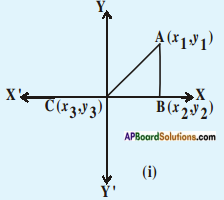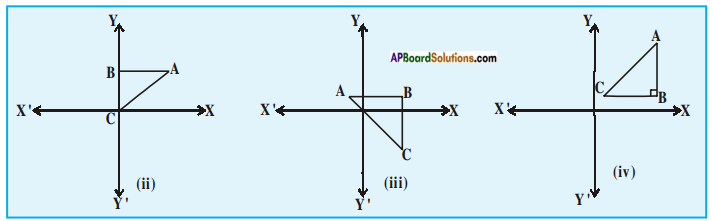i) Given △AOB where A(x1, y1), B(x2, y2) and C(x3, y3)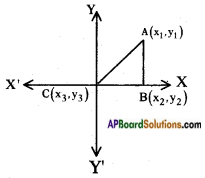But we know that the origin O is (0, 0) which is given as C.
$$\overline{\mathrm{CB}}$$ = x2 – x3 = x2 – 0 = x2
$$\overline{\mathrm{AB}}$$ = y1 – y2 = y1 – 0 = y1
∴ Area of △ABC = $$\frac{1}{2}$$ × base × height
= $$\frac{1}{2}$$x2y1ii)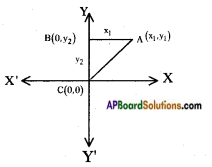A(x1, y1) = (x1, y1)
B(x2, y2) = (0, y2)
C(x3, y3) = (0, 0)
∴ Area of △ABC = $$\frac{1}{2}$$ × base × height
= $$\frac{1}{2}$$ × y2 × x1
= $$\frac{1}{2}|$$x1y2| sq. units.

iii)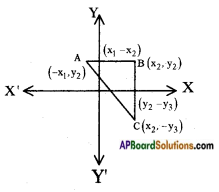∴ AB = x1 – x2
BC = y2 – y3
A(x1, y1) = (-x1, y1)
B(x2, y2) = (x2, y2)
C(x3, y3) = (x3, -y3)
∴ Area of △ABC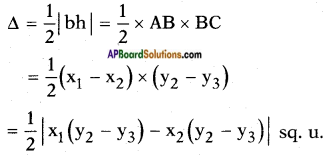iv)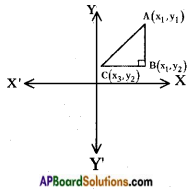AB = (y2 – y1)
BC = (x1 – x3)
A(x1, y1) = (x1, y1)
B(x2, y2) = (x1, y2)
C(x3, y3) = (x3, y2)
∴ Area of △ABC = $$\frac{1}{2}$$ × base × height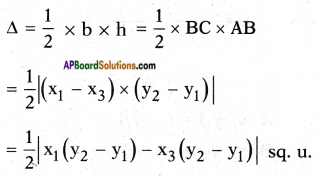Question 2.
Find the area of the triangle formed by the following points    (Page No. 181)
i) (2, 0), (1, 2), (1, 6)
Take the third point as (-1, 6).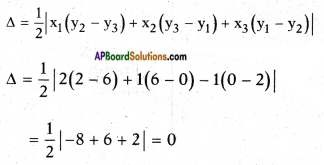ii) (3, 1), (5, 0), (1, 2)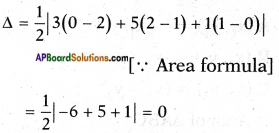iii) (-1.5, 3), (6, 2), (-3, 4)
Take the second point as (6, -2)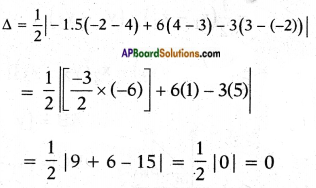Question 3.
What do you observe?          (Page No. 181)
We observe that the area formed by above all triangles is zero.

Question 4.
Plot these points on three different graphs. What do you observe?      (Page No. 181)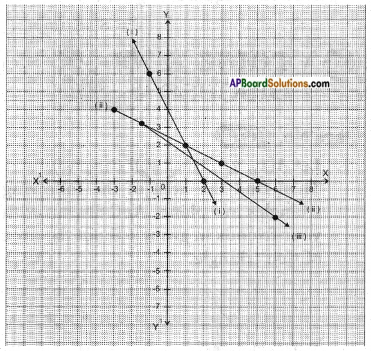We observe that the points are collinear.

Question 5.
Can we have a triangle having area zero square units area?    (Page No. 181)
No.

Question 6.
What does it mean?      (Page No. 181)
If the area of the triangle formed by any three points is zero, it means the points are collinear.Do these

Question 1.
Plot these points on the coordinate axis and join them:
Which gives a straight line? Which is not? Why?    (Page No. 185)
i) A(1, 2), B(-3, 4), C(7, -1)
Points ABC gives a straight line.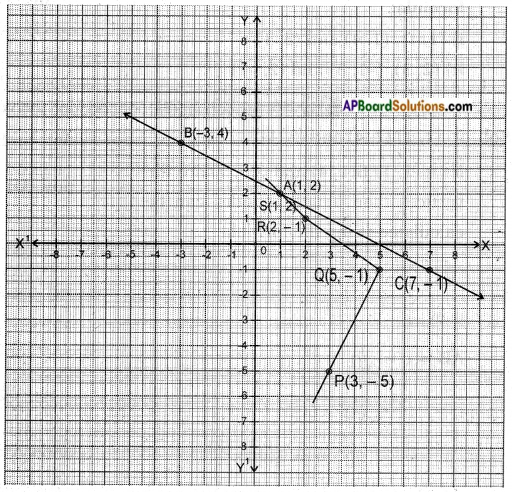ii) P(3, -5), Q(5, -1), R(2, 1), S(1, 2)
Points PQRS doesn’t give a straight line.

Question 2.
Find the slope of $$\overleftrightarrow{\mathbf{A B}}$$ with the given end points,      (Page No. 188)
i) A(4, -6), B(7, 2)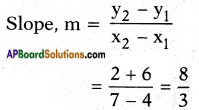ii) A(8, -4), B(-4, 8)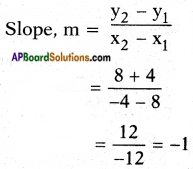iii) A(-2, -5), B(l, -7)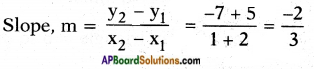Try these

(Page No. 188)

Question 1.
Find the slope of AB with the points lying on
i) A(2, 1), B(2, 6)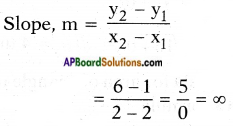Question 2.
A(-4, 2), B(-4, -2)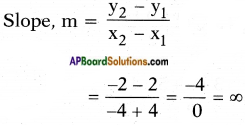Question 3.
A(-2, 8), B(-2, -2)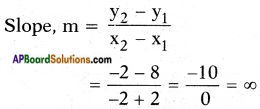Question 4.
Justify that the line AB line segment formed by given points is parallel to Y-axis. What can you say about their slope? Why?
In the above problem, all points are of the form (K, y) where K is a fixed number and y is a variable.
∴ All lines in the above problem are parallel to Y – axis. Slope of lines parallel to y – axis are not defined.

Think & Discuss

Question 1.
Does y = x + 7 represent a straight line? Draw the line on the coordinate plane. At which point does this line intersect Y – axis?
How much angle does it make with X – axis? Discuss with your friends.        (Page No. 185)